# Lights Out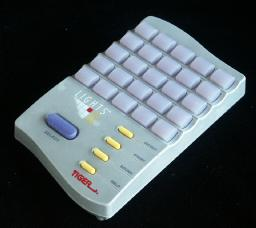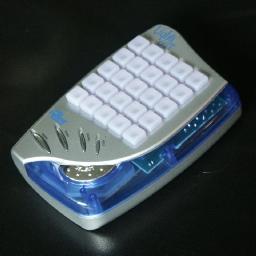# Lights Out Classic

This electronic puzzle by Tiger Toys consists of a 5×5 grid of buttons which also have lights in them. By pressing a button, its light and those of the (non-diagonally) adjacent buttons will change (switch on if it was off, and vice versa). Given a pattern of lights, you have to switch them all off by pressing the correct buttons. Not only that, but you have to do it in as few moves as possible.

This is the one of the few non-trivial puzzles I know in which the order that the moves are performed is unimportant (i.e. the puzzle positions form an Abelian group). This means that it is not necessary to press any button more than once during the solution because we could change the order of the moves so that repetitions occur together. Since pressing a button twice will not change anything, a button need only be pressed at most once.
Another puzzle of this kind is the Rubik's Clock puzzle, or the Orbix puzzle (type 1).

The classic version of Lights Out has recently been given a facelift, and now has a more curvy design. The new version has exactly the same game play, and the same built-in puzzles as the original version.

The first electronic version of this game was called the XL-25, was produced by Vulcan Electronics Ltd. in 1983, and it was invented by László Mérõ. It not only could play the now standard game where each button changes a cross of lights, but also played a variant where each button changes its own light and those lights which are a chess knight's move away. An even older electronic game called Merlin had a similar game called Magic Square which was played on a 3x3 grid, but its moves were slightly different. More on these variants later on.

The Tiger version of Lights Out was patented in the US on 23 May 1995 (US patents 5,417,425, 5,573,245, 5,603,500). I have not yet been able to find any patents for the Merlin, but the XL-25 was patented on 21 July 1983, WO83/02399 (and 27 October 1983, WO83/03691).

Amusingly the puzzle can momentarily be seen in the film "Drive" with Mark Dacascos. Near the end you can see the Lights Out puzzle used as an electronic keypad to the left of a big metal door.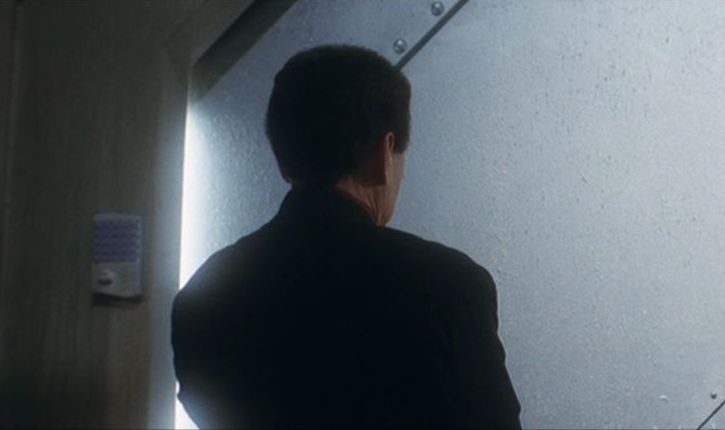## Quiet patterns:

You would expect that all 25 buttons are needed, but actually we only need to use 23 of the 25 buttons to solve any position. To show this, we can use the following 'quiet' patterns, button patterns which when pressed will leave the lights unchanged.
 X . X . X X . X . X . . . . . X . X . X X . X . X
 X X . X X . . . . . X X . X X . . . . . X X . X X
 . X X X . X . X . X X X . X X X . X . X . X X X .

Note the pattern on the right is simply a combination of the other two. These quiet patterns can be used to decrease the number of moves in a solution, but more of this later.

Using these patterns, we can see why we need never press the first two buttons. Instead of pressing them, we can use the following button patterns to get the same effect:

 . . X . X X . X . X . . . . . X . X . X X . X . X
 . . X X . X . X . X X X . X X X . X . X . X X X .

## The number of positions:

From the above it follows that there are at most 223 positions (because there are only 223 button patterns which can solve it). As there are no further quiet patterns, all these button patterns have different effects on the lights. Therefore there are 223 = 8,388,608 possible light patterns attainable.

It is actually easy to test if a position can be solved. Look at the first quiet pattern, and consider the lights marked there. Any of the 25 button presses will change an even number of the marked lights. Therefore, the only solvable patterns have an even number of the marked lights switched on. The same holds for the second pattern. If both these sets of lights have an even number of them lit, then the pattern is solvable.

I have calculated the how many positions there are for each number of buttons pressed and each number of lights switched on. There is no position that needs more than 15 button presses to solve. Note that where before the quiet patterns were used so as not to press the first two buttons, now the quiet patterns are used to minimise the total number of moves - see the section on solving in the minimal number of moves. The results can be seen in this table.

## Solution of Lights Out (Classic):

Number the rows 1-5, the columns A-E.

1. For each light on row 1, press the button beneath it on row 2 to turn the light off. This way row 1 is completely unlit.
2. Repeat step a for rows 2-4, so that now you only have lights on row 5. This is usually called 'chasing the lights'.
3. If the light at A5 is on, press D1 and E1.
If the light at B5 is on, press B1 and E1.
If the light at C5 is on, press D1.
4. Repeat steps a-b, chasing the lights down and it will be magically solved.

This method will not solve the puzzle in the shortest possible way, but it is very simple.

If your browser has JavaScript, then you can play the standard Lights Out game:

## Solving in the minimal number of moves:

To solve a puzzle in the minimal number of moves, first use steps a-c above to find out which buttons should be pressed in the top row. Restart the puzzle, and begin by pressing those top row buttons and chase the lights down. If this did not solve it in the minimal number of moves, then you should try to combine your solution with each of the three quiet patterns. One of these will give a minimal solution.

An example:
Look at the patterns below. Suppose you have the light pattern on the left. Chasing the lights produces the next light pattern. From the algorithm above, you know that if you pushed B1 and D1 first (E1 cancels) then you would have solved it. If you solve the first pattern again using this fact, then you will have used the button pattern shown. This uses 14 moves. If you combine this with the first quiet pattern, you get a worse solution (16 moves), with the second quiet pattern you get a 10 move solution, but with the third and final quiet pattern you get the optimal 8 move solution shown.

Light pattern
 . X . X . . X X X X X X . X X X X X X X X X X X X
After chasing
 . . . . . . . . . . . . . . . . . . . . X X . X X
First solution
 . X . X . X . . . X X X X X . . . X X X . X . X X
Optimal solution
 . . X . . . . X . . . . X . X X . . X . . . X . X

# Mini Lights Out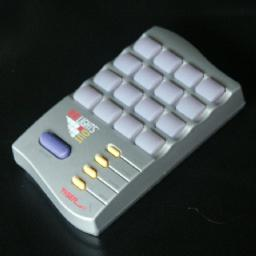This version is looks like a normal Lights Out played on a 4×4 square. The main difference is that the board has no edges - the left and right columns are considered to be adjacent, as are the top and bottom rows. Every light therefore has exactly 4 neighbours, and so every move changes exactly 5 lights. For example, pressing the top-left button changes its own light, the light below, the light to the right, the light to the 'left' (the top right corner), and the light 'above' (the bottom left corner). This mathematics of this variant is exactly the same, though light chasing is not really possible.

The Mini Lights Out also has a second type of game, the Lit-only game. This is just the same as the normal games, except that you are only allowed to press buttons that are lit. Pressing an unlit button has no effect. This restriction makes it somewhat more difficult.

## The number of positions:

This game has no quiet patterns, which means that each light can be changed without affecting the others. Therefore all 216 light patterns are solvable.

## Solution to the Mini Lights Out:

This is an easy puzzle once you know the following two facts:
1. To change an individual light, press it and its four neighbours.
2. To know whether you need to press a button or not, check its own light and the neighbouring lights. If an odd number of these 5 lights are switched on, then the button needs to be pressed, otherwise it does not.

The following solution then suggests itself:
a. Use fact 2 above on all the buttons in the middle two rows.
b. For each light that is on in row 2, press the button above it in row 1.
c. For each light that is on in row 3, press the button below it in row 4.

## Lit Only games:

The lit-only game is solved in nearly the same manner as the normal game. Simply figure out whether any of the lit buttons need to be pressed, and then press them. If any unlit button needs to be pressed, you will need to delay pressing it until other button presses have lit it. Occasionally all buttons that remain in the solution are unlit, so then some lit button has to be pressed first to allow you to move on. Later that same button will have to be pressed again, since it wouldn't have been part of the solution if you hadn't been forced to press it the first time. This situation can usually be avoided.

I have proved that any position that is solvable in a normal variation of the lights-out is also solvable as the lit-only game. The proof of this is given on the Lights Out Mathematics page.

If your browser has JavaScript, then you can play the Mini Lights Out game:

# Lights Out 2000## Description of Lights Out 2000:

This version is very similar to the classic version, i.e. a 5×5 grid of buttons with lights which you have to switch off in as few moves as possible. The difference lies in the fact that now the lights are not just on or off, but have 3 states - off, red, or green. When a button is pressed the same lights change as before, but now they go from off to red to green and then off again.

If you buy only one Lights-Out game, I would recommend this one, because not only does it do this 3-state puzzle, it also incorporates the classic Lights Out puzzle, and has an action game and a 2 player game as well. For a short time in 2001 this version was sold without the 2000 tag, but now Tiger has re-released the original classic Lights-Out in a new design that looks very similar to the Lights-Out 2000. The Lights-Out 2000 can be easily recognised by the obvious tiger-paw arrangement of the buttons.

The mathematics of the 3-state puzzle is much the same. The move order is unimportant, and pressing a button three times has no effect, so no button has to be pressed more than twice to solve any position. All the mathematics works the same way, except that the arithmetic is done modulo 3 instead of modulo 2.

## Quiet patterns:

This puzzle has five types of quiet patterns:
(grey means press once, black twice)

 1 . . . 2 2 2 . 1 1 1 2 . 1 2 1 1 . 2 2 . 2 . 1 .
 1 . 1 . . 2 1 2 2 . 2 1 . 2 1 1 2 1 1 . 1 1 2 2 1
 . 1 2 2 2 2 . 1 . 2 1 2 . 1 2 1 . 2 . 1 1 1 1 2 .
 1 . 2 . 1 2 . 1 . 2 . . . . . 1 . 2 . 1 2 . 1 . 2
 1 1 . 2 2 1 1 . 2 2 . . . . . 2 2 . 1 1 2 2 . 1 1

These patterns can occur in any orientation, in mirror image, or with the colours swapped. Together with the trivial quiet pattern (no buttons pressed at all) this leads to 27=33 quiet patterns. This suggests that this time there are three buttons that need never be used.

## The number of positions:

Three buttons need never be used to solve the puzzle, so 22 buttons are enough. Each button can be pressed up to two times, giving a total of 322 = 31,381,059,609 positions for the lights.

The built-in puzzles need between 5 and 24 moves to solve, and this seems to imply that any position can be solved in at most 24 moves. I have now verified this by calculating the number of possible positions for each number of buttons pressed. The results can be seen on the tables page.

## Solution to Lights Out 2000:

1. For each light on row 1, press the button beneath it on row 2 once or twice to turn the light off. This way row 1 is completely unlit.
2. Repeat step a for rows 2-4, so that now you only have lights on row 5. This is usually called 'chasing the lights'.
3. If the light at A5 is red then press B1 twice, if it is green press it once.
If the light at B5 is red then press A1 and B1 twice, if it is green press them once.
4. Repeat steps a-b, chasing the lights down and it will be magically solved.

To get a minimal solution, the same method can be used as for the classic version, except that there are now 26 quiet positions to check.

The built-in puzzle problems can be solved using the above method, or you can simply look up the solution on this page, which also includes the solutions to the built-in puzzles of the classic game.

If your browser has JavaScript, then you can play the Lights Out 2000 game:

# Lights Out Cube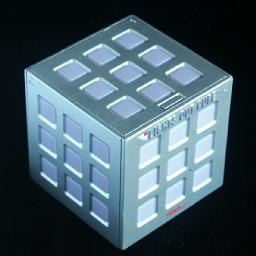This version is like the classic Lights Out (all lights are either on or off) except that it is played on a 3×3×3 cube. Whereas the classic Lights Out has edges, the cube does not, so each light always has 4 neighbours, and every button press changes exactly 5 lights. This interesting variant can be solved using similar methods, except that light chasing is very different and much more complicated.

## Quiet patterns:

The cube has 26 = 64 quiet patterns. This is a lot, but this is partly because the cube has more symmetry than a square. There are actually 8 types of quiet pattern, which can be applied in several orientations. They are shown below. By applying the first of these patterns in several orientations, you can also generate the other 7 patterns.
 . X . . . . . X .
 . . . X . X X . X
 X X X . . . . X .
 . . . X . X X . X
 X X X . . . . X .
 . X . . . . . X .
 . . . . . . . . .
 X . X . . . X . X
 X . X . . . X . X
 X . X . . . X . X
 X . X . . . X . X
 . . . . . . . . .
 X X . X . X . X X
 X . . . . . X . .
 . . X . . . . . X
 . . X . . . . . X
 X . . . . . X . .
 . X X X . X X X .
 . X . X . X . X .
 X X X X . X X X X
 X X X X . X X X X
 X X X X . X X X X
 X X X X . X X X X
 . X . X . X . X .
 X . . X . X . . X
 X . . X . X . . X
 X X . . . . . X X
 . . X X . X X . .
 . X X . . . X X .
 . . X X . X X . .
 X . X X . X X . X
 X X X . . . . X .
 X . X X . X . . .
 X X X . . . . X .
 X . X X . X . . .
 X . X X . X X . X
 . . X . . . X . .
 X X . X . X X X .
 X X . X . X X X .
 . X X X . X . X X
 . X X X . X . X X
 X . . . . . . . X
 . X . X . X . X .
 . X . X . X . X .
 . X . X . X . X .
 . X . X . X . X .
 . X . X . X . X .
 . X . X . X . X .

## The number of positions:

There are 26 quiet patterns, which means that the 54 buttons do not generate 254 patterns, but 254-6 = 248 = 281,474,976,710,656 positions. The manual incorrectly calls this 'over 200 quadrillion', as it is a mere 281 trillion (in the American sense of the word).

I have calculated the number of possible positions for each number of buttons pressed. It turns out that no positions need more than 30 moves to solve. The results can be seen on the tables page.

## Solution to the Lights Out Cube:

 1 2 3 4 5 6 7 8 9
 10 11 12 22 23 24 34 35 36
 13 14 15 25 26 27 37 38 39
 16 17 18 28 29 30 40 41 42
 19 20 21 31 32 33 43 44 45
 46 47 48 49 50 51 52 53 54

1. Number the buttons/lights as shown above (which is different to the numbering in the manual).
2. First you must simplify the position by chasing the lights:
If 5 is lit, then press it to switch it off.
If 1, 2 or 3 are lit, then press the buttons below them, i.e. 21, 20, or 19, to switch them off.
If 7, 8 or 9 are lit, then press the buttons below them, i.e. 13, 14, or 15, to switch them off.
If 4 or 6 are lit, then press the buttons below them, i.e. 11 or 17, to switch them off.
If any of 10-21 are lit, then press the buttons below them, i.e. 22-33 to switch them off.
If any of 22-33 are lit, then press the buttons below them, i.e. 34-45 to switch them off.
If 35 or 41 are lit, then press the buttons below them, i.e. 49 or 51, to switch them off.
If 37, 38, or 39 are lit, then press the bottoms below them, i.e. 46, 47, or 48, to switch them off.
If 43, 44, or 45 are lit, then press the bottoms below them, i.e. 54, 53, or 52, to switch them off.
If 50 is lit, then press it to switch it off.
3. There are now only lights on in the bottom face or at 34, 36, 40, 42.
If 40 is lit, then press the buttons 3 and 4.
If 36 is lit, then press the buttons 4 and 16.
If 46 is lit, then press the buttons 16 and 10.
If 34 is lit, then press the buttons 10 and 1.
If 47 is lit, then press the buttons 4.
If 49 is lit, then press the buttons 2, 12 and 16.
4. Repeat step b, chasing the lights down and it will be magically solved.

If your browser has JavaScript, then you can play the Lights Out Cube game:

# Lights Out Deluxe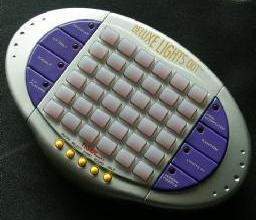The 6×6 Light Out version was manufactured by Tiger and sold as the Lights Out Deluxe. This version allowed you to play several different versions of the game. The normal game has moves where each button press changes its own light and the four lights horizontally and vertically adjacent to it. A game with a different type of move is also possible - each button press changes its own light and the four lights diagonally adjacent to it. Thus instead of a normal cross (+) you now do a diagonal cross (×).

Not only are two move types available, there are also three puzzle types, the normal game and two others. In the Lit-Only game you are only allowed to press lit buttons, and pressing an unlit button has no effect. In the Toggle game you must alternately press lit and unlit buttons. All three game types can be played with either move type. For all 6 move/game combinations there are 150 built-in puzzles.

## The number of positions:

There are no quiet patterns for either move type, so all 236 = 68,719,476,736 possible light patterns are possible.

## Solution for Lights Out Deluxe (move type +):

Number the rows 1-6, the columns A-F.
1. For each light on row 1, press the button beneath it on row 2 to turn the light off. This way row 1 is completely unlit.
2. Repeat step a for rows 2-5, so that now you only have lights on row 6.
3. If the light at A6 is on, press A1 and C1.
If the light at B6 is on, press D1.
If the light at C6 is on, press A1 and E1.
If the light at D6 is on, press B1 and F1.
If the light at E6 is on, press C1.
If the light at F6 is on, press D1 and F1.
4. Repeat steps a-b, chasing the lights down and it will be magically solved.

As there are no quiet patterns on this board size, this solution will always use the minimal number of moves if you cancel out duplicate moves from the two chases (or restart the game once you know which buttons to press on the top row).

## Solution for Lights Out Deluxe (move type ×):

Number the rows 1-6, the columns A-F.
1. Press a combination of buttons on row 2 such that the lights on row 1 are switched off. This seems tricky, but is quite easy once you know how. First switch off lights A1, C1, E1 from left to right as follows:
1. If A1 is on then press B2.
2. If C1 is on then press D2.
3. If E1 is on then press F2.
Next switch off lights A2, A4, A6 from right to left as follows:
1. If F1 is on then press E2.
2. If D1 is on then press C2.
3. If B1 is on then press A2.
Now row 1 is completely unlit.
2. Repeat step a for rows 2-5, so that now you only have lights on row 6.
3. If the light at A6 is on, press F1.
If the light at B6 is on, press E1.
If the light at C6 is on, press D1.
If the light at D6 is on, press C1.
If the light at E6 is on, press B1.
If the light at F6 is on, press A1.
Note how the button pattern to press on the top row is the mirror image of the light pattern on the bottom row.
4. Repeat steps a-b, chasing the lights down and it will be magically solved.

For this move type there are again no quiet patterns, so this solution will always use the minimal number of moves if you cancel out duplicate moves from the two chases (or restart the game once you know which buttons to press on the top row).

An interesting thing about the diagonal cross shape is that if you were to colour the game square like a chess board, then each move only affects squares of one colour. This shows that this puzzle therefore actually consists of two independent puzzles that are solved simultaneously. In the regular or lit-only game, you can actually solve one of these sets completely before tackling the other.

## Lit Only and Toggle games:

The lit-only game is solved in nearly the same manner as the normal game. Chase the lights down until the only lights left are those on the bottom row. This light-chasing might be a little tricky if the button you wish to press is not lit, but at this point it does not matter if you do more moves than necessary. Simply do whatever moves you need to do on the unsolved rows to light up the button you want to press, and then press it. Eventually you will have only lights on the bottom row, and you can find out which buttons were supposed to be pressed on the top row, just like in the normal solutions above. Restart the game and solve it again, starting with the button presses on the first row. You may need to use a few extra moves on the rows below to enable you to press a button that is unlit. If so, try to look ahead so that you will not press buttons that you will have to press again later on.

I have proved that any position that is solvable in a normal lights-out game is also solvable in the lit-only game. The proof of this is given on the Lights Out Mathematics page.

The Toggle game is slightly more difficult. Often the method as described for the lit-only game will solve the puzzle with few enough extra moves to allow you to pass to the next level. Sometimes it is more tricky though, and then you will first have to solve the position using the regular game rules. As you do so, make a note of which buttons form the solution. Then play it in the Toggle manner, choosing the solution buttons in such an order that they are alternatively lit and unlit. Note that the built-in puzzles always have an order in which it is possible, i.e. it is not necessary to do moves other than those involved in the normal solution. Puzzles with an even number of moves therefore start with an unlit button-press, those with an odd number of moves with a lit button-press. The theory behind the Toggle game can be found on the Lights Out Mathematics page.

The 6×150 built-in puzzle problems can be solved using the above methods. You can also look up the solutions on this page.

If your browser has JavaScript, then you can play Lights Out Deluxe game:

# Gamze Lights Out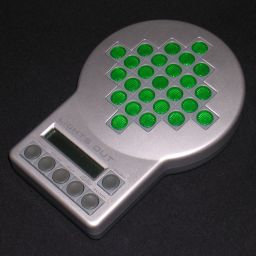A new version of Lights Out is currently being made by Gamze. It seems to be made under license because it uses Tiger's trademarked name and lists the patent number on the back. The board of this version is a diamond shaped grid of 25 squares, with rows and columns of lengths 1, 3, 5, 7, 5, 3, and 1. There are two move types, either the standard vertical cross (+) or the diagonal cross (×), similar to the Deluxe. It also has a choice of the classic game or the Lit Only game.

## The number of positions in Gamze Lights Out, + move:

In this version every light can be changed individually by certain button presses, so there are no quiet patterns and the number of possible positions is 225 = 33,554,432.

## Solution for Gamze Lights Out, + move:

1. Try to solve as many of the lights as possible by a few button presses.
2. Find a light that you want to change.
3. Find a pattern in the top row in the table below that matches that light. You may need to rotate or reflect the pattern to get a match.
4. Press the buttons in the corresponding pattern in the bottom row of the table. This changes the light.
5. Repeat b-d until all the lights are correct.
 . . . . . . . . . . . . X . . . . . . . . . . . .
 . . . . . . . . . . . . . . . . . . . X . . . . .
 . . . . . . . . . . . . . . . . . . . . . . X . .
 . . . . . . . . . . . . . . . . . . X . . . . . .
 . . . . . . . . . . . . . . . . . . . . . . . X .
 . . . . . . . . . . . . . . . . . . . . . . . . X
 X X X X X . . . X X X . X . X X X . . . X X X X X
 . . . X . . X X X . . X . . . . X X . X X X . X .
 X X X X X . . . X X X . X . X X X . X . X . . . .
 . X . X X X . X X . . X . X . . . . . . . X X X X
 X X X . X . X X . X X X X . X X . X X X . X . . .
 X X X X X . . . X X X . X . X X X . X . X . . . X

These patterns can also be used another way since the game is a symmetric one (i.e. buttons mutually affect each other). This gives a method to solve the game in the minimum number of moves.

1. Choose any button for which you want to determine if it needs to be pressed or not.
2. Find a pattern in the top row that matches that button. You may need to rotate or reflect the pattern to get a match.
3. Look at the corresponding pattern of lights given in the bottom row. If an odd number of the marked lights are incorrect then the button needs to be pressed, otherwise it does not.
4. Repeat a-c for all the buttons in turn.

It is not necessary to memorize all six patterns to be able to solve it optimally. Imagine the board marked like a chessboard, alternating black or white. Pressing a square of one colour will not affect other squares of the same colour. Therefore if you solve only the white button presses squares with the above method, then the black button presses needed to solve the rest are simply those black squares with their lights off. Therefore you only need to memorize three of the six patterns (either patterns 1,2,3 or patterns 4,5, and 6) to be able to solve this puzzle.

## Quiet patterns for the × move:

If you colour the grid like a chessboard, alternating black and white squares, it is clear that the × move only affects squares of one colour. The black squares and the white squares are two indepentently solvable Lights Out games. One set forms a 4×4 square grid, the other a 3×3 grid. The former has four types of quiet patterns, patterns button pushes that have no effect on the lights. The 3×3 grid has no such patterns.

 X X . . X . X . . . . . . . . . X . X . . X . . X
 . X . X . . X . . X . . . X . . X . . . . . . X X
 X X . . X . X . . X . X . X . X . . X . X . . X X
 . X . X X . . . X . . . . . . . X . . . X X . X .

These patterns can occur in any orientation, or in mirror image. Together with the trivial quiet pattern (no buttons pressed at all) this leads to 16=24 quiet patterns. This suggests that there are four buttons that need never be used.

## The number of positions in Gamze Lights Out, × move:

There are 24 quiet patterns, which means that the 225 possible button patterns fall into sets of 24 that have the same effect on the lights. Therefore there are only 225/24 light patterns, i.e. only 225-4 = 221 = 2,097,152 positions.

## Solution for Gamze Lights Out, × move:

First we shall solve the 3×3 subproblem.

1. Imagine the 3×3 subgrid is numbered 1 to 9 in the obvious manner.
2. To see whether edge button 8 has to be pressed or not, look at lights 1, 2, 3, and 5, i.e. the three lights on the opposite side of the square together with the centre one. If one or three of those four lights are on, then press button 8. If an even number of them is on, do not press it.
3. Repeat step b with the other three edge buttons.
4. The five remaining buttons, the corners and the centre, are now simple to solve. Of those five buttons, press the lit ones and do not press the unlit ones. The 3×3 grid should now be all off.

Now we solve the 4×4 subproblem.

1. Orient the puzzle so that the top row of the 4×4 grid has as few lights on as possible.
2. If pressing a top corner would switch off three lights, then do so.
3. Switch off the top row completely by pressing the button directly below any lit top row square.
4. Switch off the second row the same way but pressing the buttons on the third row.
5. Switch off the third row the same way but pressing the buttons on the bottom row. When you have finished, the bottom row will automatically be solved too.

The 4×4 part of the solution will not necessarily be optimal, but will take at most 4 moves too many. This is well within the margin that the game allows.

# Merlin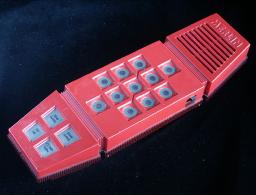In 1978 Parker Brothers made an electronic game called Merlin. It could play various games, like Tic-Tac-Toe and Blackjack. One of the games was Magic Square, and it is probably the first ever type of Lights Out game. It is played on a 3×3 board, and the effects of button presses are as follows:

• Corner Button: Changes the four lights of the 2×2 square in that corner.
• Edge Button: Changes the three lights along that side of the board.
• Centre Button: Changes 5 lights, the centre one and the 4 adjacent ones (as in the normal Lights Out)

The aim of the game is to switch on all the lights except for the one in the centre, i.e. create a ring of lights.

Merlin has been reissued in a smaller more managable version, and it still has a Magic Square game. However, the moves are different from the original. They are now exactly the same as the standard Lights Out, i.e. each button press changes its own light as well as the orthogonally adjacent ones. It has simply become a 3×3 Lights out.

## The number of positions in the original Merlin Magic Square:

Every light can be changed individually by certain button presses, so there are no quiet patterns and the number of possible positions is 29 = 512.

## Solution for the Original Merlin Magic Square Game:

The squares are numbered 1-9 in the normal way.

1. For edge button 8, look at the lights 4, 5, 6, 7, 8, and 9 which form a 2×3 rectangle along that edge of the board. If an even number of lights in this rectangle are on, then press button 8. Do the same for each of the other edge buttons, using the rectangle along those sides instead.
2. Press any unlit corner buttons.
3. If the centre light 5 is on, then press it to switch it off.

## The number of positions in the reissued Merlin Magic Square:

As with the original, every light can be changed individually by certain button presses, so there are no quiet patterns and the number of possible positions is 29 = 512.

## Solution for the reissued Merlin Magic Square Game:

The squares are numbered 1-9 in the normal way.

1. For edge button 8, look at the lights 1, 2, 3, and 5 which form a T on the opposite edge of the board. If an even number of these lights are on, then press button 8. Do the same for each of the other edge buttons, using the T-shape along those opposite sides instead.
2. Press any unlit corner buttons.
3. If the centre light 5 is on, then press it to switch it off.

# XL 25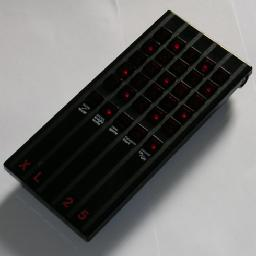Around 1983 Vulcan Electronics Ltd made an electronic game called the XL 25. It could play two types of game. It played the standard Lights-Out game (although actually the aim was to switch all the lights on) and also a variant where each button changes its own light and those lights which are a chess knight's move away.

The XL-25 was patented by David Ildiko, Laszlo Meroe, and Ferenc Szatmari, number WO83/02399, filed 16 January 1982, published 4 January 1983.

The Lights-Out variant need not be discussed further. The solution for the classic Lights Out can be used, except that on/off is swapped, i.e. when you chase lights you have to switch each row on instead of off. Below only the Knight's Game is discussed.

## The number of positions in the XL 25 Knight's Game:

In the knight's game, every light can be changed individually by certain button presses, so there are no quiet patterns and the number of possible positions is 225 = 33,554,432.

## Solution for the XL-25 Knight's game:

1. Try to solve as many of the lights as possible by a few button presses.
2. Find a light that you want to change.
3. Find a pattern in the top row in the table below that matches that light. You may need to rotate or reflect the pattern to get a match.
4. Press the buttons in the corresponding pattern in the bottom row of the table. This changes the light.
5. Repeat b-d until all the lights are correct.
 X . . . . . . . . . . . . . . . . . . . . . . . .
 . X . . . . . . . . . . . . . . . . . . . . . . .
 . . X . . . . . . . . . . . . . . . . . . . . . .
 . . . . . . X . . . . . . . . . . . . . . . . . .
 . . . . . . . X . . . . . . . . . . . . . . . . .
 . . . . . . . . . . . . X . . . . . . . . . . . .
 X X . . X X . X X . . X . . . . X . . X X . . X .
 X . . X . . X . X X X X X . X X X X . X . . X X X
 . . X . . X X . X X X . . . X X . . . X . X . X .
 . X X X X X X . . X X . . X . X . X . . X X . . .
 X . . . X X . X . X . . . . . . X . X . . X . X .
 . X . X . X . . . X . . X . . X . . . X . X . X .

These patterns can also be used another way since the game is a symmetric one (i.e. buttons mutually affect each other). This gives a method to solve the game in the minimum number of moves.

1. Choose any button for which you want to determine if it needs to be pressed or not.
2. Find a pattern in the top row that matches that button. You may need to rotate or reflect the pattern to get a match.
3. Look at the corresponding pattern of lights given in the bottom row. If an odd number of the marked lights are incorrect then the button needs to be pressed, otherwise it does not.
4. Repeat a-c for all the buttons in turn.

It is not necessary to memorize all six patterns to be able to solve it. Imagine the board marked like a chessboard, alternating black or white. Pressing a square of one colour will not affect squares of the same colour. Therefore if you solve only the white button presses with the above method, then the black button presses needed to solve the rest are simply those black squares with their lights off. Therefore you only need to memorize the second and fifth pattern (or alternatively the other four patterns) to be able to solve this puzzle.

## Further variants

There are a many variations of the classic Lights Out game possible. The most obvious changes you can make have already been encountered in the Deluxe - changing the board size and the move type. The game can of course be played on a square or rectangular board of any size. The basic solving strategy does not change, as you can still chase lights down to the last row. Each board size will have its own quiet patterns though (or possibly none at all). The effect of a move could be changed, i.e. pressing a button gives a pattern other than a vertical cross, for example the diagonal cross of the Deluxe, or the Knight's Game of the XL 25. If a button changes lights that are not adjacent but further away then light chasing becomes quite tricky if not impossible.

The behaviour at the edges of the board can be changed too. The columns of buttons on the left and right sides could be considered to be adjacent, i.e. as if the board were curved around into a cylinder. If the top and bottom sides are also considered adjacent, the board is in effect a torus. On this board it is more difficult to chase lights effectively because you will not end up with a single row of lights but two rows of lights. This was seen in the Mini Lights Out.

The game can also be played on something other than a recangular grid. One such radically different version is the Orbix, which is played on the 12 faces of a dodecahedron. Any graph can be used as the playing field for this game.

Lastly you can change the number of colours. This was seen in Lights Out 2000, which has 3 states, but you could use more colours too. It is even possible to mix different numbers of colours for example by having some buttons use only 2 states while others have 3, though I have not yet seen such a variant. Usually repeated button presses cycle the affected lights through all possible states. One unusual variant is TileToggle which has 4 colours and two types of move.

If your browser has JavaScript, then you can play some Lights Out variants by clicking the link below. The board can be any rectangular size from 3×3 to 10×10, can be a rectangle, cylinder or torus, and the move shape can be changed too. It is a little slow however when you change the board size or move type, because it has to find the new move matrix and invert it, as explained in the mathematics section.

## JavaScript Lights Out Variants.

I have also written a Java applet that allows you to play Lights Out on any graph. You can create any graph to play on, and also share it with everyone by uploading it to the server.